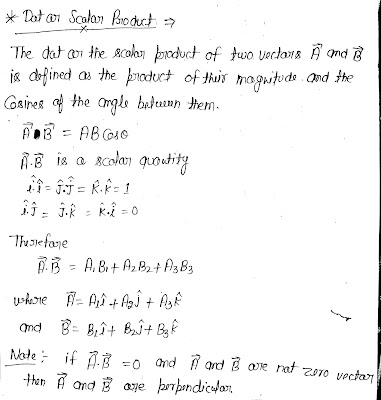## Scalar and Cross Product

These are the most important product of our physics because they are widely used in our all subjects.
so do not forget to cover this topic before you start your preparation. so here is the video and notes for this topic. i hope you will love it.Scalar and Cross Product

### Dot or Scalar Product

The dot or scalar product of two vectors A and B is defined as the product of their magnitude and the cosines of angle between them.

A.B = AB Cos(theta)

Here A.B is a scalar quantity.Scalar or Dot Product

### Cross or Vector Product

The cross or Vector Product of two vectors A and B taken in that order is defined as the AB sin(theta)unit vector.

A cross B = AB sin(theta) unit vector

where theta is the angle between A and B.Cross or Vector Product